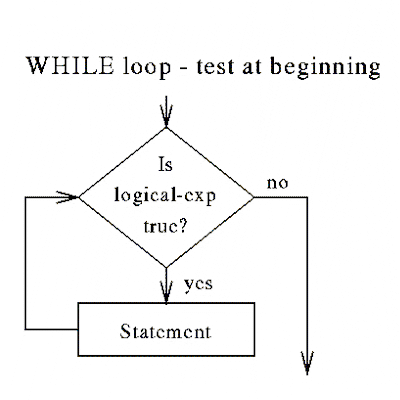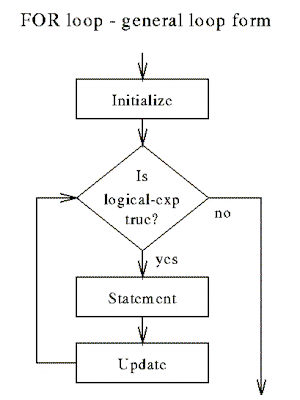Computers in Engineering - 308-208 Lecture 15
Computers in Engineering - 308-208

# Lesson 15 - Learning Goals

## 15.4 Learn how to use the C for loop

Is logical expression true ?

If YES, go through the loop statements.

If NO, do not execute the while loop.EXAMPLE :

```/* How many digits ? */

ndigit = 1;
number = 10000 ;
while ( number >= 10 ) {
number = number / 10 ;
ndigit = ndigit + 1 ;
}

```

DO LOOP - test at end

Do the loop once ( execute loop statements ).

Then, is logical expression true ?

If YES, go through the loop statements again.

If NO, exit the loop.

```/* How many digits ? */

ndigit = 0;
number = 10000 ;
do
{
number = number / 10 ;
ndigit = ndigit + 1 ;
} while ( number != 0 ) ;
```

SCANF

```#include <stdio.h>
main( )
{
int d1, d2, d3, d4, d5, d6, d7 ;

while ( scanf("%1d%1d%1d%1d%1d%1d%1d",
&d1, &d2, &d3, &d4, &d5, &d6, &d7) = = 7 )
{
printf (" Reverse : %1d%1d%1d%1d%1d%1d%1d \n",
d7, d6, d5, d4, d3, d2, d1 ) ;
}

}
```

EXAMPLE :

***************************************************

Input                                 Output

```1234567           Reverse : 7654321

7 6 5 4 3 2 1     Reverse : 1234567

0987654 2345678   Reverse : 4567890

Reverse : 8765432

1234567890        Reverse : 7654321

1234              Reverse : 4321098

```

SCANF

```#include <stdio.h>

main( )
{

char cmd [ 15 ] ;
int i ;
i = scanf (" %s ", cmd ) ; /* No & before cmd because cmd
is an array */

if ( i = = -1 ) printf (" Blank input \n ") ;
else printf ("The input is %s \n ", cmd) ;
}

Input : 23456

Output : Blank input

```

scanf returns a function value equal to the number of good arguments.

It returns 0 if there is no input, and -1 if EOF (end of file)

FOR LOOP - general loop form

for ( Initialize ; logical expression ; update variable )

Is logical expression true ?

If YES, go through the loop statements.

Then, Update the initial condition

Is logical expression true ?

If YES, go through the loop statements.

Then, Update the initial condition

Is logical expression true ?

.

.

.

If NO, exit the loop.EXAMPLE :

```/* Execute the same statement 10 times */

for( i=0 ; i < 10 ; i++ )
{
j = j + 1 ;
}

```

FOR LOOP IN C

```for ( init, init, . . . , init ;
expression ;
update, update, . . . , update )
{
statements ;
}

```

EXAMPLE :

```for ( ndigit = 1; number >= 10 ; ndigit += 1 )
{
number = number/10 ;
}

```

DIFFERENCES FROM FORTRAN :

• No trip count calculated ahead of time
• Update not restricted to increments
• No special '' loop counter ''

On to the next lecture